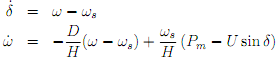## Compute the transfer function from the input, Electrical Engineering

Assignment Help:

In this project we will consider the control of a synchronous generator supplying electricity to the grid. We will focus on the problem of frequency stability. The frequency at which the generator rotates is directly proportional to the frequency of the currents and voltages in the power grid. Hence, keeping the rotational frequency constant is of fundamental importance to the correct operation of the grid. A simpli ed model to study the stability of a single generator connected to the grid is the so called swing equation given by:In this model !s is the synchronous rotational velocity 2 50 rad/s. The constant D = 5 represents the damping induced by mechanical and electrical losses and the constant H has the value 4. The term Pm = 1=2 represents the mechanical power that is transformed by the generator into electrical power and the term U sin  represents the electrical power and describes the e ect the grid has on the generator. We will take U as the input since we can change its value by the voltage across the excitation coil in the generator's rotor.

A synchronous generator, when operating in steady state, maintains a constant angular velocity achieved by matching the supplied mechanical power Pm with the power lost through dissipation H (is) and the electrical power U sin . When a fault occurs and a transmission line is tripped (opened) there is a sudden change in the electrical power and some generators will accelerate while other generators will decelerate. Once the fault is cleared and the transmission line is re-closed, di erent generators will be rotating at di erent angular velocities and voltages and currents in the power grid will no longer be sinusoids with a frequency of 50 Hz.

The objective of this project is to design a controller that will resume the steady state operation of the generator at 50 Hz. Please justify all your answers including relevant plots if necessary.

#### Quartus, I have a project due tomorrow, i have exhuasted all my resources, ...

I have a project due tomorrow, i have exhuasted all my resources, and cannot understand how to do the project, it is VHDL coding in Quartus program, i can send the assignment if yo

#### State lenz''s law, Lenz's law states: 'The direction of an induced e.m....

Lenz's law states: 'The direction of an induced e.m.f. is always like that it tends to set up a current opposing the motion or the change of flux responsible for inducing that

#### Microprocessor, highest priority interrupt in 8085 microprocessor

highest priority interrupt in 8085 microprocessor

#### Power electronics, waveform for diode with RLC load

waveform for diode with RLC load

#### Dual trace and dual beam cro, what is difference between dual trace and dua...

what is difference between dual trace and dual beam cro?

#### #title thyristor, what is finger voltage in terms of thyristor

what is finger voltage in terms of thyristor

#### Name some application of serial communication, Name some application where ...

Name some application where you see use of serial communication. Within the world of serial communications, there are two different equipments are: DTE stand for Data Termin

#### Protection against overload current, explain the operation principle

explain the operation principle

#### Electrical/electronic engineering project, i need a project creating in ele...

i need a project creating in electronic/electrical engineering at HNC level, 3000 words Harvard referencing. it can be about anything in this field or i can give a suggestion

#### Find the ratio of amplifier gain, The input to the satellite system of Figu...

The input to the satellite system of Figure 1 is a step function θ c (t) = 5u(t) in degrees. As a result, the satellite angle θ(t) varies sinusoidally at a frequency of 10 cycles p